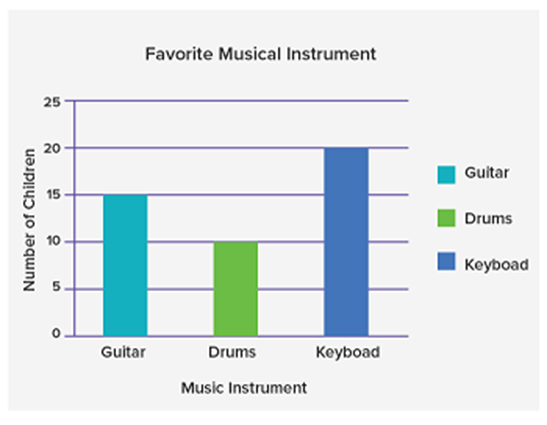Mathematics
Easy

Question

# The number of children choose guitar is ________.## 15102025Hint:

## The correct answer is: 15

### Now we know that a graph is made up of vertices, or points, and edges, or lines connecting those vertices. Graphs come in a wide variety of forms in discrete mathematics.The heights of the rectangular bars in a bar graph, which displays complete data, are proportionate to the values they indicate.There are two types of a bar graph: Vertical Bar Graph Horizontal Bar Graph Although the bars in bar graphs can be plotted either horizontally or vertically, the vertical bar graph is the one that is most frequently used.Now we have given a bar graph which is a Vertical one. In that graph, there are 3 bars, Guitar, Drums, and Keyboards.So we have asked how many children choose a guitar. So from the graph, we can see that the bar of the guitar is touching the y-axis at 15.So 15 students choose a guitar.

Here we used the concept of bar graphs and understood are different types of bar graphs. So 15 students choose a guitar. Although the bars in bar graphs can be plotted either horizontally or vertically, the vertical bar graph is the one that is most frequently used. So 15 students choose a guitar.

### Related Questions to study#### With Turito Foundation.#### Get an Expert Advice From Turito.